# Numpy Matrices and Matplotlib new stuff¶

Create a list of numbers and plot them.

In :
42

Out:
42
In :
grades = [70.2, 66, 80.3, 95.2, 80, 91]

In :
grades

Out:
[70.2, 66, 80.3, 95.2, 80, 91, 'hi']
In :
print(grades)

[70.2, 66, 80.3, 95.2, 80, 91, 'hi']

In :
import matplotlib.pyplot as plt
%matplotlib inline

In :
plt.plot(grades);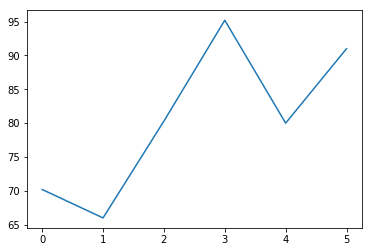In :
plt.plot(grades,'-o')
plt.xlabel('Assignment')
plt.ylabel('Grade')

Out:
<matplotlib.text.Text at 0x7f2bb51fd048>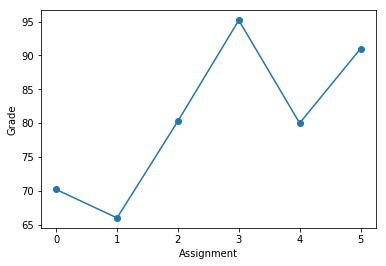How about data showing miles-per-gallon for cars manufactured in particular years?

In :
mpg = [22, 25, 20, 32, 19, 42, 28]
mpg

Out:
[22, 25, 20, 32, 19, 42, 28]

But, what about the years?

In :
years = [2010, 2012, 2013, 2012, 1999, 2014, 2004]
years

Out:
[2010, 2012, 2013, 2012, 1999, 2014, 2004]
In :
plt.plot(mpg)

Out:
[<matplotlib.lines.Line2D at 0x7f2bc11fec50>]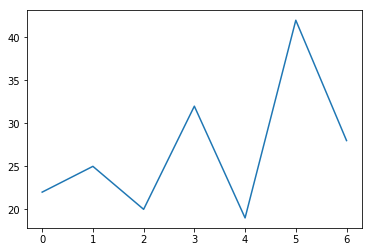Not really what we want. Want to plot mpg on y axis and year on x axis.

In :
plt.plot(years, mpg)

Out:
[<matplotlib.lines.Line2D at 0x7f2bb5143be0>]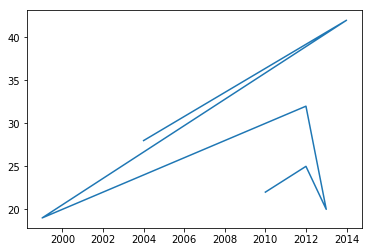In :
years

Out:
[2010, 2012, 2013, 2012, 1999, 2014, 2004]
In :
plt.plot(years, mpg,'o')

Out:
[<matplotlib.lines.Line2D at 0x7f2bb505a240>]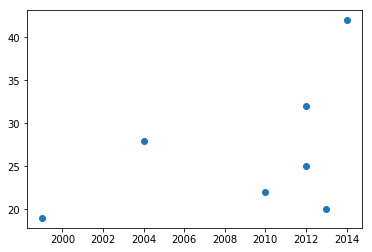Is there a linear relationship here? How can I draw a line through this graph and play with its parameters?

In :
def line(x, slope, yintercept):
return x * slope + yintercept

In :
s = 3
yint = -5990
line(1998, s, yint)

Out:
4
In :
line(2014, s, yint)

Out:
52
In :
for y in years:
print(y)

2010
2012
2013
2012
1999
2014
2004

In :
ys = []
for y in years:
ys.append(line(y, s, yint))
ys

Out:
[40, 46, 49, 46, 7, 52, 22]
In :
plt.plot(years, mpg, 'o')
plt.plot(years, ys)

Out:
[<matplotlib.lines.Line2D at 0x7f2bb52290f0>]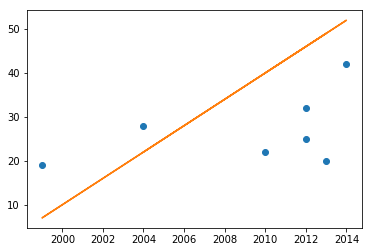Wow! That's a pain! First of all, let's jump into the wonderful world of matrices. We can often replace for loops with single matrix calculations.

In :
import numpy as np

In :
mpg = np.array([22, 25, 20, 32, 19, 42, 28])
mpg

Out:
array([22, 25, 20, 32, 19, 42, 28])
In :
years = np.array([2010, 2012, 2013, 2012, 1999, 2014, 2004])
years

Out:
array([2010, 2012, 2013, 2012, 1999, 2014, 2004])

Watch this! Our function, line, just returns x * slope + yintercept. If each of these variables actually contain numpy arrays of the same shape, the operations will automatically be applied component-wise.

In :
line(years, s, yint)

Out:
array([40, 46, 49, 46,  7, 52, 22])
In :
plt.plot(years, mpg, 'o')
plt.plot(years, line(years, s, yint))

Out:
[<matplotlib.lines.Line2D at 0x7f2bb4e6e8d0>]Works, but remember the x values are not in order. If all values did not fall in a line, we would not see a nice function plot. We can make this more obvious by adding some noise to each estimated y value.

In :
np.random.rand(5)

Out:
array([ 0.78438157,  0.40085623,  0.56611822,  0.46844871,  0.79880259])
In [ ]:
plt.plot(years, line(years, s, yint) + np.random.rand?

In :
years.shape

Out:
(7,)
In :
np.random.rand(7) * 10

Out:
array([ 6.58075188,  4.86190617,  6.69217929,  7.33451778,  5.49714337,
7.25997226,  1.85575394])
In :
plt.plot(years, mpg, 'o')
plt.plot(years, line(years, s, yint) + np.random.rand(7)*10)

Out:
[<matplotlib.lines.Line2D at 0x7f2bc00d7550>]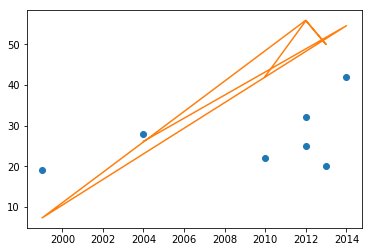Now it is clear we need to order our data by year.

In :
print(np.sort(years))
years

[1999 2004 2010 2012 2012 2013 2014]

Out:
array([2010, 2012, 2013, 2012, 1999, 2014, 2004])
In :
yearsSorted = np.sort(years)
plt.plot(yearsSorted, line(yearsSorted, s, yint) + np.random.rand(7)*10)

Out:
[<matplotlib.lines.Line2D at 0x7f2bb4c06ef0>]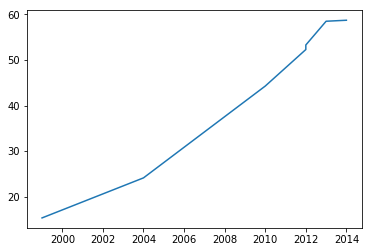In [ ]:
yearsSorted = np.sort(years)
plt.plot(yearsSorted, line(yearsSorted, s, yint), 'o-')


Now it is clear we need to order our data by year. But we have two (parallel) arrays. Can find order of indices that will arrange years in ascending order, and apply the indices to the years.

In :
order = np.argsort(years)
order

Out:
array([4, 6, 0, 1, 3, 2, 5])
In :
years

Out:
array([2010, 2012, 2013, 2012, 1999, 2014, 2004])
In :
years[0:3]

Out:
array([2010, 2012, 2013])
In :
years, mpg

Out:
(array([2010, 2012, 2013, 2012, 1999, 2014, 2004]),
array([22, 25, 20, 32, 19, 42, 28]))
In :
years[order], mpg[order]

Out:
(array([1999, 2004, 2010, 2012, 2012, 2013, 2014]),
array([19, 28, 22, 25, 32, 20, 42]))

It is too easy to make errors with parallel arrays. Instead, let's combine our year-mpg samples into a matrix with each row being one sample.

In :
np.stack((years, mpg))

Out:
array([[2010, 2012, 2013, 2012, 1999, 2014, 2004],
[  22,   25,   20,   32,   19,   42,   28]])
In [ ]:
np.stack?

In :
np.stack((years, mpg), axis=1)

Out:
array([[2010,   22],
[2012,   25],
[2013,   20],
[2012,   32],
[1999,   19],
[2014,   42],
[2004,   28]])
In :
data = np.stack((years, mpg), axis=1)

In :
data[0, 0]

Out:
2010
In :
data[0, :]

Out:
array([2010,   22])
In :
data[:, 0]

Out:
array([2010, 2012, 2013, 2012, 1999, 2014, 2004])
In :
plt.plot(data[:, 0], data[:, 1])

Out:
[<matplotlib.lines.Line2D at 0x7f2bb4b2bf28>]In [ ]:
order = np.sort?

In [ ]:
order = np.sort

In :
order = np.argsort(data[:, 0])
order

Out:
array([4, 6, 0, 1, 3, 2, 5])
In :
data[order, :]

Out:
array([[1999,   19],
[2004,   28],
[2010,   22],
[2012,   25],
[2012,   32],
[2013,   20],
[2014,   42]])
In :
data = data[np.argsort(data[: ,0]), :]

In :
data

Out:
array([[1999,   19],
[2004,   28],
[2010,   22],
[2012,   25],
[2012,   32],
[2013,   20],
[2014,   42]])
In :
plt.plot(data[:, 0], data[:, 1], 'o');In :
plt.plot(data[:, 0], data[:, 1], '-o');
plt.plot(data[:, 0], line(data[:, 0], s, yint));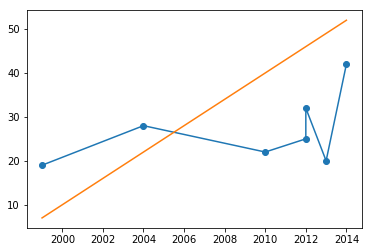Now, we have the basics of defining functions, collecting data samples in a matrix, and plotting them. Time to automate the fitting of a line to the data–linear regression!